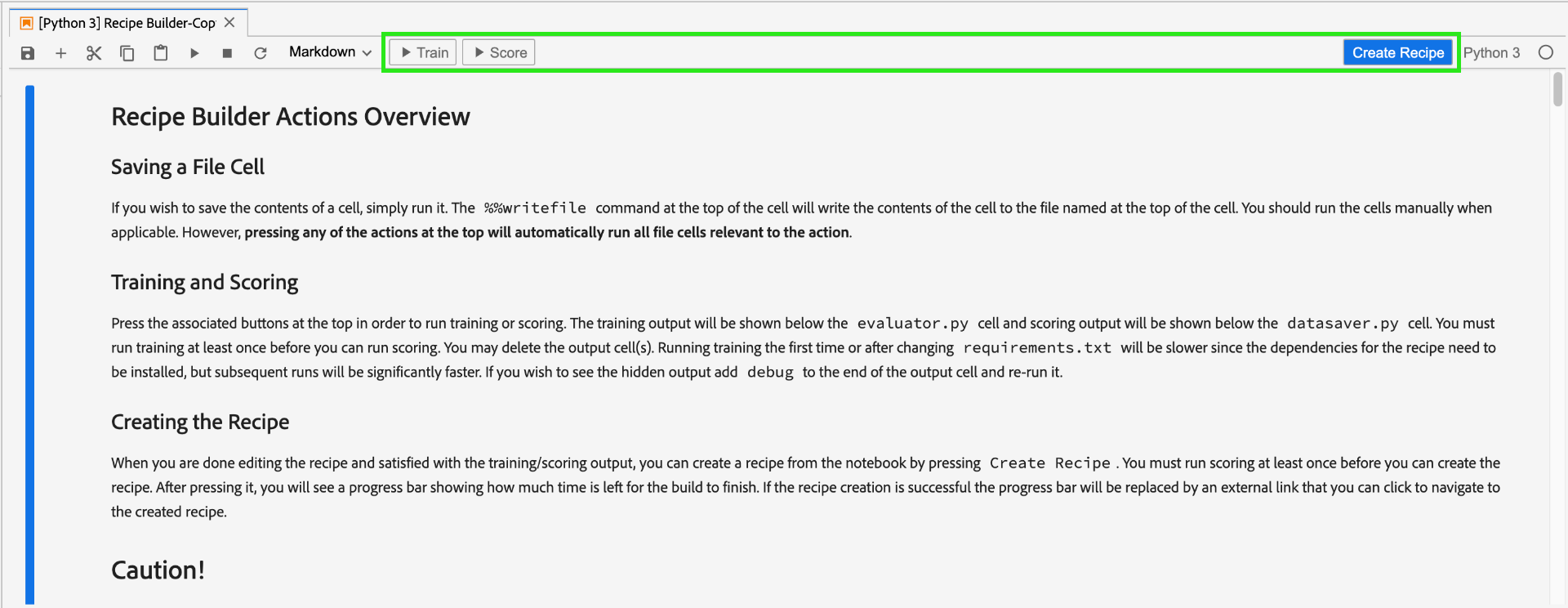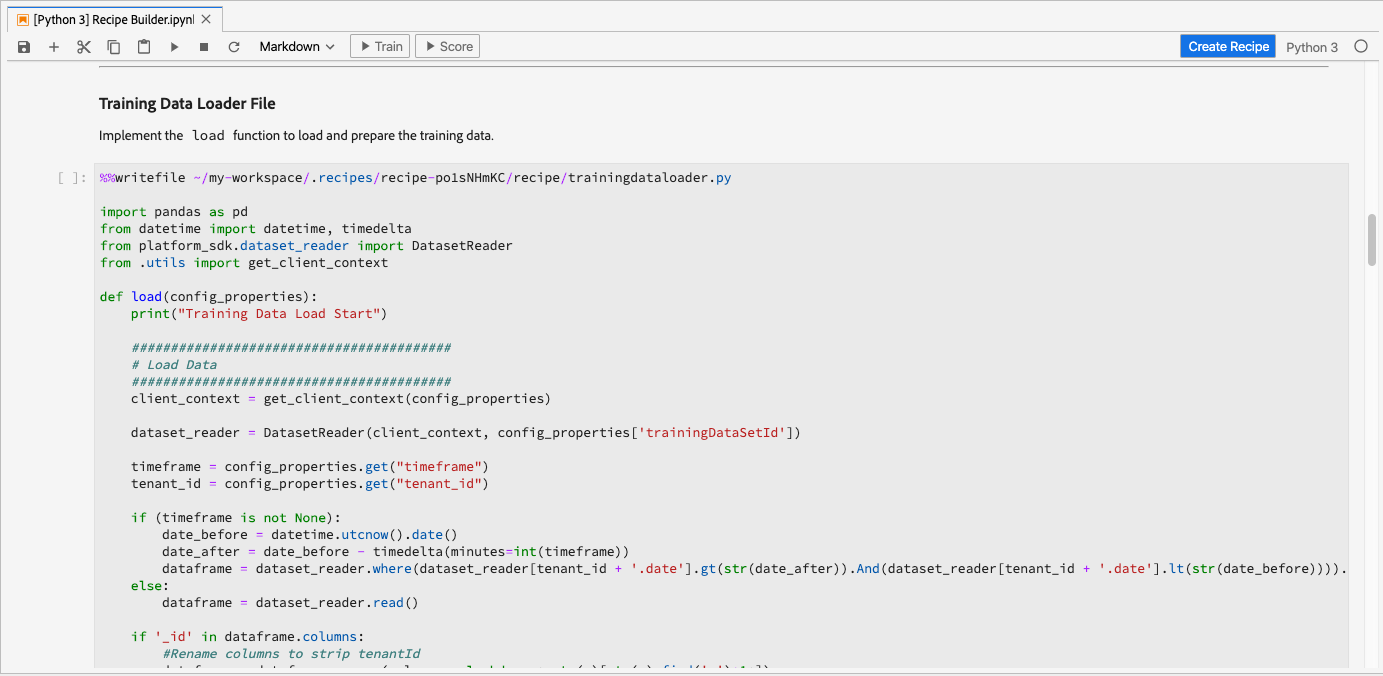# 使用Jupyter Notebooks创建菜谱

## 引入的概念：

• 模型： 模型是机器学习方法的实例，该方法使用历史数据和配置进行培训，以便针对业务用例进行解决。
• 培训： 培训是从标记数据中学习模式和洞察的过程。
• 评分： 评分是使用经过培训的模型从数据生成洞察的过程。

## 开始使用JupyterLab笔记本环境

Recipe Builder笔记本可以在笔记本内运行培训和评分。 这使您能够灵活地在对培训和评分数据运行实验之间更改其`train()``score()`方法。 一旦您对培训和评分的输出感到满意，您就可以创建一个菜谱，在Data Science Workspace中使用笔记本创建菜谱功能，该功能内置于菜谱生成器笔记本中。

Recipe Builder笔记本支持使用所有文件格式，但当前“创建菜谱”功能仅支持Python。## Recipe Builder笔记本入门

### 要求文件

``````python=3.6.7
scikit-learn
pandas
numpy
data_access_sdk_python
``````

### 配置文件

• `trainingDataSetId`
• `ACP_DSW_TRAINING_XDM_SCHEMA`
• `scoringDataSetId`
• `ACP_DSW_SCORING_RESULTS_XDM_SCHEMA`
• `scoringResultsDataSetId`• `ML_FRAMEWORK_IMS_USER_CLIENT_ID`
• `ML_FRAMEWORK_IMS_TOKEN`
• `ML_FRAMEWORK_IMS_ML_TOKEN`
• `ML_FRAMEWORK_IMS_TENANT_ID`

## 培训数据加载器

• 从Platform加载数据
• 数据准备和功能工程

### 外部源

``````df = pd.read_csv(data)
``````

``````df = pd.read_json(data)
``````

### 从Platform SDK

`from platform_sdk.dataset_reader import DatasetReader`

``````def load(config_properties):

#########################################
#########################################
client_context = get_client_context(config_properties)

timeframe = config_properties.get("timeframe")
tenant_id = config_properties.get("tenant_id")
``````

• `ML_FRAMEWORK_IMS_USER_CLIENT_ID`
• `ML_FRAMEWORK_IMS_TOKEN`
• `ML_FRAMEWORK_IMS_ML_TOKEN`
• `ML_FRAMEWORK_IMS_TENANT_ID`

### 数据准备和功能工程

``````#########################################
# Data Preparation/Feature Engineering
#########################################
dataframe.date = pd.to_datetime(dataframe.date)
dataframe['week'] = dataframe.date.dt.week
dataframe['year'] = dataframe.date.dt.year

dataframe = pd.concat([dataframe, pd.get_dummies(dataframe['storeType'])], axis=1)
dataframe.drop('storeType', axis=1, inplace=True)
dataframe['isHoliday'] = dataframe['isHoliday'].astype(int)

dataframe['weeklySalesLag'] = dataframe.shift(45)['weeklySales']
dataframe['weeklySalesDiff'] = (dataframe['weeklySales'] - dataframe['weeklySalesLag']) / dataframe['weeklySalesLag']
dataframe.dropna(0, inplace=True)

dataframe = dataframe.set_index(dataframe.date)
dataframe.drop('date', axis=1, inplace=True)
``````

• 添加`week``year`
• `storeType`转换为指示符变量
• `isHoliday`转换为数字变量
• 偏移`weeklySales`以获得未来和过去的销售值
• 按日期将数据拆分为`train``val`数据集

`load()`函数应以`train``val`数据集作为输出。

### 评分数据加载器

``````def load(config_properties):

#########################################
#########################################
client_context = get_client_context(config_properties)

timeframe = config_properties.get("timeframe")
tenant_id = config_properties.get("tenant_id")
``````

``````    #########################################
# Data Preparation/Feature Engineering
#########################################
if '_id' in dataframe.columns:
#Rename columns to strip tenantId
dataframe = dataframe.rename(columns = lambda x : str(x)[str(x).find('.')+1:])
#Drop id, eventType and timestamp
dataframe.drop(['_id', 'eventType', 'timestamp'], axis=1, inplace=True)

dataframe.date = pd.to_datetime(dataframe.date)
dataframe['week'] = dataframe.date.dt.week
dataframe['year'] = dataframe.date.dt.year

dataframe = pd.concat([dataframe, pd.get_dummies(dataframe['storeType'])], axis=1)
dataframe.drop('storeType', axis=1, inplace=True)
dataframe['isHoliday'] = dataframe['isHoliday'].astype(int)

dataframe['weeklySalesLag'] = dataframe.shift(45)['weeklySales']
dataframe['weeklySalesDiff'] = (dataframe['weeklySales'] - dataframe['weeklySalesLag']) / dataframe['weeklySalesLag']
dataframe.dropna(0, inplace=True)

dataframe = dataframe.set_index(dataframe.date)
dataframe.drop('date', axis=1, inplace=True)

return dataframe
``````

``````df['weeklySalesAhead'] = df.shift(-45)['weeklySales']
``````

``````df['weeklySalesLag'] = df.shift(45)['weeklySales']
df['weeklySalesDiff'] = (df['weeklySales'] - df['weeklySalesLag']) / df['weeklySalesLag']
``````

``````df.dropna(0, inplace=True)
``````

### 管道文件

`pipeline.py`文件包含培训和评分逻辑。

### 培训

`train()`函数应包括培训模型并返回培训模型。 不同型号的一些示例可在scikit-learn用户指南文档中找到。

``````def train(configProperties, data):

print("Train Start")

#########################################
# Extract fields from configProperties
#########################################
learning_rate = float(configProperties['learning_rate'])
n_estimators = int(configProperties['n_estimators'])
max_depth = int(configProperties['max_depth'])

#########################################
# Fit model
#########################################

seed = 1234
n_estimators=n_estimators,
max_depth=max_depth,
random_state=seed)

model.fit(X_train, y_train)

print("Train Complete")

return model
``````

### 评分

`score()`函数应包含评分算法并返回一个度量，以指示模型执行的成功程度。 `score()`函数使用评分数据集标签和训练的模型来生成一组预测特征。 然后，将这些预测值与评分数据集中的实际特征进行比较。 在此示例中，`score()`函数使用经过训练的模型来使用评分数据集中的标签来预测特征。 返回预测特征。

``````def score(configProperties, data, model):

print("Score Start")

y_pred = model.predict(X_test)

data['prediction'] = y_pred
data = data[['store', 'prediction']].reset_index()
data['date'] = data['date'].astype(str)

print("Score Complete")

return data
``````

### 求值器文件

`evaluator.py`文件包含如何评估培训菜谱以及如何拆分培训数据的逻辑。 在零售销售示例中，将包含加载和准备培训数据的逻辑。 我们将浏览以下两节。

### 拆分数据集

`split()`函数如下所示。 参数中提供的数据帧将拆分为要返回的`train``val`变量。

``````def split(self, configProperties={}, dataframe=None):
train_start = '2010-02-12'
train_end = '2012-01-27'
val_start = '2012-02-03'
train = dataframe[train_start:train_end]
val = dataframe[val_start:]

return train, val
``````

### 评估已训练的模型

`evaluate()`函数在模型训练后执行，并将返回一个度量来指示模型执行的成功程度。 `evaluate()`函数使用测试数据集标签和“训练”模型来预测一组特征。 然后将这些预测值与测试数据集中的实际特征进行比较。 常见评分算法包括：

``````def evaluate(self, data=[], model={}, configProperties={}):
print ("Evaluation evaluate triggered")
y_pred = model.predict(val)
mape = np.mean(np.abs((y_actual - y_pred) / y_actual))
mae = np.mean(np.abs(y_actual - y_pred))
rmse = np.sqrt(np.mean((y_actual - y_pred) ** 2))

metric = [{"name": "MAPE", "value": mape, "valueType": "double"},
{"name": "MAE", "value": mae, "valueType": "double"},
{"name": "RMSE", "value": rmse, "valueType": "double"}]

return metric
``````

### 数据保护程序文件

`datasaver.py`文件包含`save()`函数，用于在测试评分时保存您的预测。 `save()`函数将进行预测，并使用Experience Platform Catalog API将数据写入您在`scoring.conf`文件中指定的`scoringResultsDataSetId`

``````from data_access_sdk_python.writer import DataSetWriter

def save(configProperties, prediction):
print("Datasaver Start")
print("Setting up Writer")

writer = DataSetWriter(catalog_url=catalog_url,
ingestion_url=ingestion_url,
client_id=configProperties['ML_FRAMEWORK_IMS_USER_CLIENT_ID'],
user_token=configProperties['ML_FRAMEWORK_IMS_TOKEN'],
service_token=configProperties['ML_FRAMEWORK_IMS_ML_TOKEN'])

print("Writer Configured")

writer.write(data_set_id=configProperties['scoringResultsDataSetId'],
dataframe=prediction,
ims_org=configProperties['ML_FRAMEWORK_IMS_TENANT_ID'])

print("Write Done")
print("Datasaver Finish")
print(prediction)
``````

## 创建菜谱• 请勿删除任何文件单元格
• 请勿编辑文件单元格顶部的`%%writefile`
• 请勿同时在不同笔记本中创建菜谱